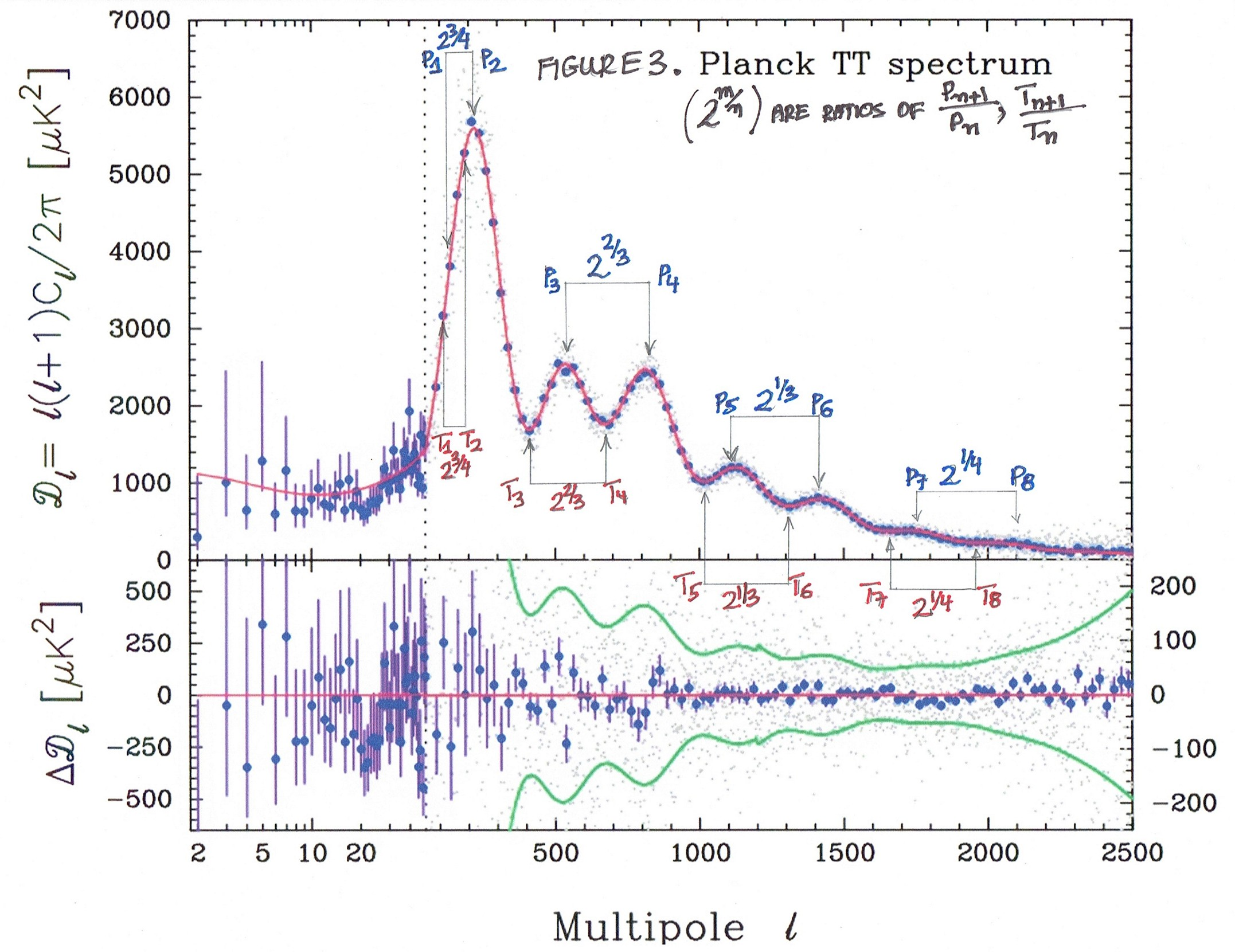6 June 2020 AD; St Norbert (1134)

25 November 2020 AD; St Catherine of Alexandria (307)

In this article, I will present the sounds created at the same time as the Universe was created. To get these sounds (Music) we need the Cosmic Microwave Background Power Spectrum. This fact was made possible only recently, thanks to the satellites such as Planck or WMAP, etc.

First, when you look at CMB (Cosmic Microwave Background) Power Spectrum you see a graph of a wavy function with Crests (Peaks) and Troughs. This is the amplitude of a function (on the Y-axis) if you want to keep things simple. We are not really interested in that - all we need are the Crests (or Peaks) and Troughs. What we need is the stuff on the X-axis. After Fourier Transform (flat geometry) and Laplace Transform (Spherical Geometry), and other complicated stuff, the astronomers get the Spherical Harmonics and then Multipole Moment l. Multipole Moment l is what we are interested in - and this is on X-axis. Let me quote someone (no name, just User Name) from Stackexchange.

"ll is a label for the spherical harmonics, and can be compared to the wave number in plane waves: it is a measure (inversely proportional to) the size of perturbations. Like the wavenumber is inversely proportional to wavelength.

You are quite right that sound waves are involved: Just like sound travelling as density waves in the air here on Earth, the photon-baryon fluid (which were coupled before decoupling) are influenced by such waves."

Now, these spherical harmonics as a Multipole Moment l may be treated as a wavenumber. Another quote:

"In the physical sciences, the wavenumber (also wave number) is the spatial frequency of a wave, either in cycles per unit distance or radians per unit distance. It can be envisaged as the number of waves that exist over a specified distance (analogous to the frequency being the number of cycles or radians per unit time)."

But we want the Frequency of sounds. Another quote:

"Frequency is a measurement of the number of wave cycles per second. Wavenumber is the reciprocal of the wavelength multiplied by a constant. Although they look the same, the difference is that the wavenumber has nothing to do with the velocity of the wave- wavenumber is measured in cm^-1, which doesn't include a time unit."

So, one can say that the Multipole Moment l is similar to the frequency (normalized) of sound. This is what we were looking for. We have frequencies of sounds on the X-axis.

Now I can present some graphs. When you look closely at some good-quality graphs of the Power Spectrum, you can assign the frequencies for Crests and Troughs of the graph. This is an approximation of course. Fortunately, there are ways to get exact values of the frequencies - I will do it in Part II of these series of articles.

Here is Figure 1. Planck 2013 Power Spectrum and Frequencies.For each Crest (Peak) and Trough, we have two approximations - the first one from Dl, and the second number from the lower portion of the Graph - Δ Dl .

There are sixteen frequencies, eight from Peaks and eight from Troughs, and as you will see in the next articles they are all beautifully connected with each other, creating a Musical Scale.

One of the tests of the exact value of the frequencies will be what I show in Figure 2. - WMAP Power Spectrum. What I mean is - if the frequencies are correct they must include the frequency of about 13-14 in value.

Figure 2. WMAP TT Power Spectrum:And finally, to show you the perfect correlation between frequencies of Peaks and Troughs there is Figure 3. Planck TT Power Spectrum, with pairs of frequencies, and their ratios which are powers of number 2. This fact is important to derive the Music Scale and to find out all the missing frequencies of the full Music Scale. Of course, powers of two are an approximation, since this music is ruled as you might already guess by my favourite - Transcendental Function and Transcendental Constants. But, as the first step of approximation powers of two are very useful.

Figure 3. Planck TT Power Spectrum.This much for Part I of these series. In the next part, - Part II I will calculate the exact values of frequencies using powers of two and I will present the examples of the Notes and Chords playable on the piano or some other instruments. Then in Part III the Transcendental Music Scale, - the accurate one, and maybe something more in Part IV.

Comments powered by CComment